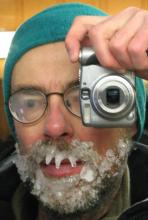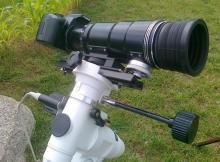# Computing error (excel or IRIS)

8 posts / 0 new
RDAA
Computing error (excel or IRIS)

Hi all!

I'm very new at this, so probably this is a very easy question ;) :

I'm following the citizensky tutorial on DSLR photometry, using IRIS as my software package and the intermediate reduction spreadsheet. I stack around 10 images for each star and measure the magnitude on the final image.

But I don't know how to compute the measurement errors. Can I use the comp. stars fit value error and the check star error to compute the standard deviation of my measurement?

If not, how can I use IRIS to calculate it? I've read that using the "stat" command gives you the sigma value of the image, but I don't know what to do with that.

Thanks!

KTCThey may be a good starting point.

RDAA
Thanks, I'll have a look at

Thanks, I'll have a look at that thread!

RDAA
SNR in IRIS

I read it, and also the new post in Matt Templeton's blog. I followed his recomendations, and in my last measurements I have reduced my error by ~3 times (from 0.04-0.06 to ~0.015). I achieved that by stacking more images (from 10 to 50), thus improving my star's SNR.

The post also links to a very interesting paper by Michael Koppelman. I found out that I was using a decent formula to estimate my errors (equation 3 in the paper). But it can be improved with the SNR.

Does somebody know how to read the SNR value in IRIS?

Thanks!

Daniel

FRFHow to determinte SNR in IRIS?

"Does somebody know how to read the SNR value in IRIS?"

This is the question I need an aswer too...
I hope someone can help.

FRFNobody can answer to this question? OK, then I rephare it.

Can anyoune propose a free software with the SNR of a DSLR image can be easily determined? Thanx!

rybiDoes somebody know how to read the SNR value in IRIS?"

[quote=FRF]

"Does somebody know how to read the SNR value in IRIS?"

This is the question I need an aswer too...
I hope someone can help.

[/quote]

I found in Romanishin's book "An Introduction to Astronomical Photometry Using CCDs" formula no 20.6 at page 118. The book is available under link: http://www.nhn.ou.edu/~wjr/research/wrccded2.pdf

S/N =Cstar / (SQRT(Cstar + 2Csky)

where: Cstar = C(star+sky) - Csky

Just make two aperture measurements in Iris:

-) one with the star in the middle of apertue --> C(star+sky),

-) second --> Csky,

and calculate.

Roger Pieri
Computing sigma and SNR

Hi !

The technique used by several of us at CS is to group the images in 5 series, stack each of the series, compute the sigma from the 5 results. The confidence level from 5 values is not very high but proved enough for that purpose. Each series should accumulate at least 60s of exposure (so small apertures are scintillation sensitive !) I often use 5 x 10 images of 10 sec with a 200mm lens F4, 50 mm aperture.

Another way is to measure all images (I do it using my automated pipe-line) and compute the sigma of each series result (mean) It's very interesting to compare it to the sigma of the five series. It's a good indicator of star or sky variability on short term.

Then I don't know any SNR calculation in IRIS but IRIS provides all terms needed. The calculation is not that simple, it involves the mean pixel background noise, the two apertures sizes, the star intensity, correlation... All calculation shall be made from electron-count, not from ADUs. You should calibrate the e-/ADU depending ISO. For a recent APS-C, CMOS, 12 Mpix, 14 bits, the 1/1 ratio is about ISO 230 (Anyhow low ISO shall be used !)

To get the background noise (read noise + sky shot noise) with IRIS I would not use the global "stat" command. The result is polluted by residues of bright stars and background gradients that are not eliminated by the median. Best is to "left click" and draw a box near the target star, in an area free of faint stars. Then "right click" and choose "statistics", you get the sigma of this area (ADU). This is the background noise per pixel.
Next go to "aperture photometry" as usual. In the result pad you get three items: the size of the foreground aperture (inner circle,pix), the size of the background aperture (pix), the computed intensity of the star (ADU). Then the calculation is not that simple, the attachement last equation provides the total noise. To get the SNR just divide the star intensity by that noise (all calculation in electrons).

Hope that helps !

Clear Skies !

Roger